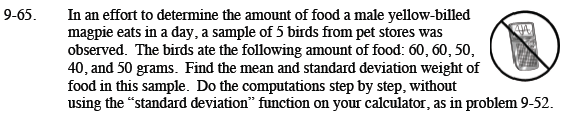### Home > CCA2 > Chapter Ch9 > Lesson 9.2.2 > Problem9-65

9-65.In an effort to determine the amount of food a male yellow-billed magpie eats in a day, a sample of 5 birds from pet stores was observed. The birds ate the following amount of food: 60, 60, 50, 40, and 50 grams. Find the mean and standard deviation weight of food in this sample. Do the computations step by step, without using the “standard deviation” function on your calculator, as in problem 9-52. Homework Help ✎mean: 52

Sample standard deviation:

$\sqrt{\frac{(60-52)^2+(60-52)^2+(50-52)^2+(40-52)^2+(50-52)^2}{5-1}}$

Note that we divide by n − 1 because this is a sample.